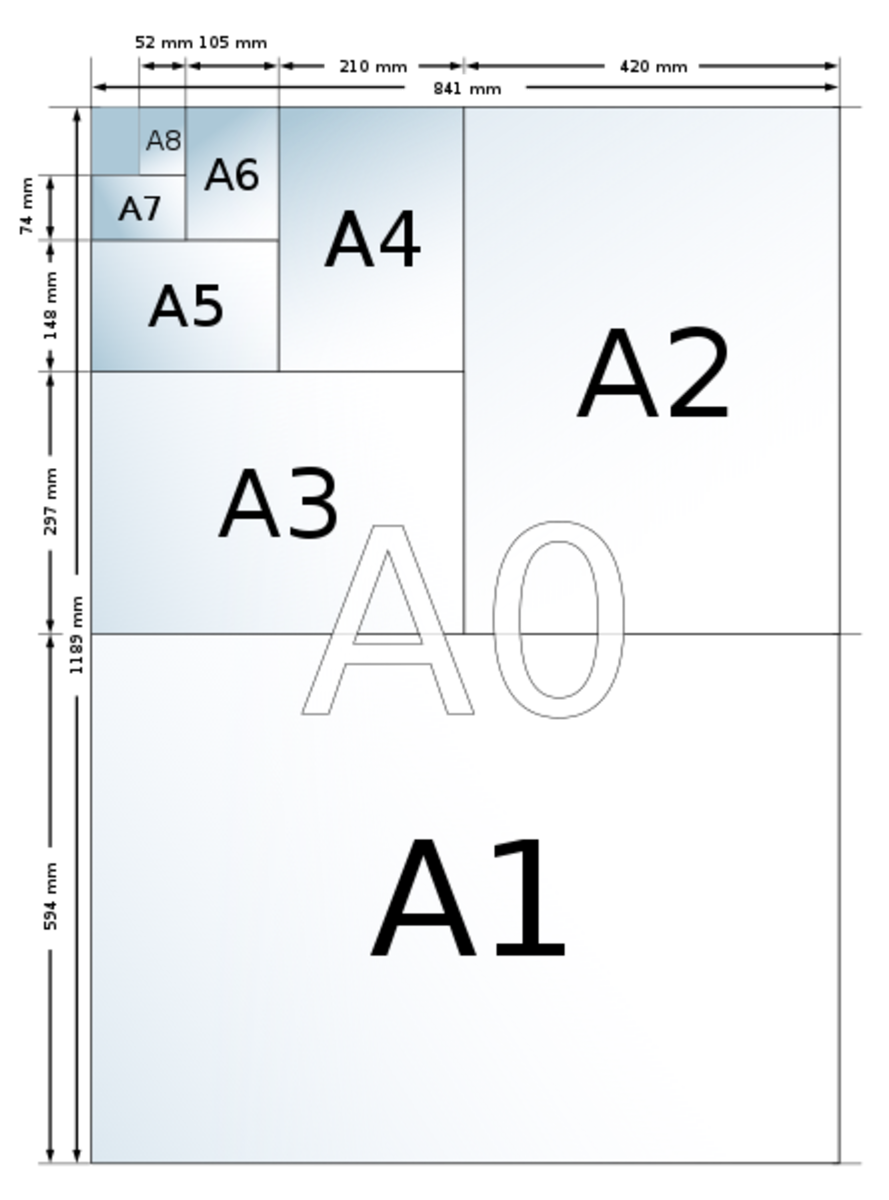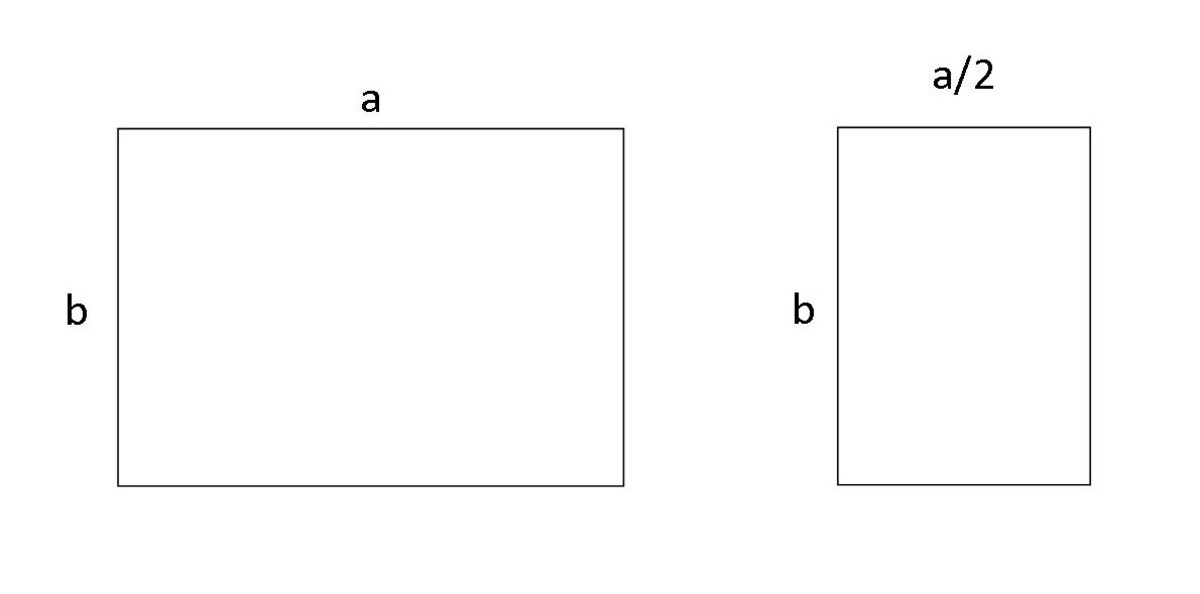# The Maths Behind A4 Paper

• Author:
• Updated date:

I am a former maths teacher and owner of DoingMaths. I love writing about maths, its applications, and fun mathematical facts.How the A-sizes of Paper CompareSven - https://commons.wikimedia.org/wiki/File:A_size_illustration.svg

## What Is A4 Paper?

A4 paper is part of the A-Series of paper sizes introduced throughout Europe in the early 20th Century and is now the official document size for most countries around the world and the United Nations organisation itself, with the main exceptions to its use being the USA and Canada.

Measuring 210 mm x 297 mm (8.3 in x 11.7 in), A4 is the most commonly used size in the A-Series, perfect for business letters and other day-to-day use, but why is it so interesting mathematically and how is it related to the other members of the A-Series? First of all, let's have a look at how it was created.

## What Happens When You Fold A4 in Half?

One useful aspect of the A-Series is what happens when you fold a sheet in half. The A-Series was created such that each time you fold a sheet in half you get a new rectangle that is mathematically similar to the old one i.e. the lengths and widths have both been scaled by the same amount. This smaller, similar rectangle is the next size in the series. For example, folding an A4 piece of paper in half gives you A5, folding an A5 in half gives you A6 and so on. Conversely, if you put two pieces of A4 together, you get A3.

For this to happen there has to be a link between the length and width of each A size. Look at the diagram below to see how this works.Folding an A-Series Piece of Paper in HalfDavid Wilson

In the photo above, we have started with a sheet of paper with dimensions a × b. If we fold this in half we get a sheet of paper with the same height, but half as wide. Its dimensions are a/2 × b.

For the smaller sheet to have the same scale as the larger sheet, the sides of the two sheets need to be in the same ratio i.e. dividing the long side by the short side gives you the same answer regardless of which rectangle you use.

Therefore we get:

a/b = b/(a/2)

a/b = 2b/a

a2 = 2b2

a = b√2

So our A-Series sheets of paper are defined by the longer side always being √2 times bigger than the small side.

This is great, but there has to be a starting point. Why does A4 have such seemingly random dimensions? The answer is in the definition of the larger size, A0.

Scroll to Continue

## How Do We Find the Measurements of A0?

As we discovered above, every size in the A-Series has a length that is √2 times the width. A0 is defined as the rectangle that fits this description and also has an area of exactly one square metre.

If we call the width of the A0 'b', its length is, therefore, b√2. As we want an area of 1 m2, we get the equation:

b × b√2 = 1

b2 √2 = 1

b2 = 1/√2

b = 1/4√2

The length, a, is √2 times this and so a =4√2.

This gives us a rectangle with dimensions 4√2 × 1/4√2 m or, rounded to the nearest millimetre, 841 mm × 1 189 mm (33.1 in × 46.8 in).

The rest of the A-Series is then defined using these numbers by halving the longer length each time, so A1 is 594 mm × 841 mm and so on. You can see the sizes of each of the A-Series sheets in the table below.

## A-Series Paper Sizes From A0 to A10

SizeWidth × Height (mm)Width × Height (in)

A0

841 × 1189

33.1 × 46.8

A1

594 × 841

23.4 × 33.1

A2

420 × 594

16.5 × 23.4

A3

297 × 420

11.7 × 16.5

A4

210 × 297

8.3 × 11.7

A5

148 × 210

5.8 × 8.3

A6

105 × 148

4.1 × 5.8

A7

74 × 105

2.9 × 4.1

A8

52 × 74

2.0 × 2.9

A9

37 × 52

1.5 × 2.0

A10

26 × 37

1.0 × 1.5

## Benefits of the A-Series

One of the main benefits of the A-Series sizes is the mathematical similarity between each size. As all dimensions are increased by the same scale factor it makes the transfer of content from one size to another very easy. For example, if you take an A4 image and enlarge it to A3, the image will maintain its proportions and not be stretched unnaturally. You get the same result if you reduce in size from one A size to another.

As each size is √2 larger than the previous one, enlarging by √2 ≈ 1.414 or 141.4% will perfectly resize A4 to A3, A3 to A2 and so on.

## The B-Series

The B-Series of paper sizes is defined similarly to the A-Series, but instead of starting with a sheet with an area of 1 m2, it starts with sheet B0 where the shortest side is 1 metre. As with the A-Series, the longest side is √2 times this or 1.414 m.

B1 is then defined as half of B0 and so on. While not as common as A-Series for stationery purposes, B-Series still has its uses. For example, US government I.D. cards are B7 size.